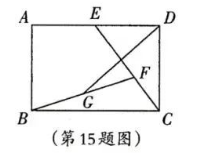A. 当 $A B=3$ 时, $D G$ 的最小值是
B. 当 $A B=4$ 时, $D G$ 的最小值是【答案】 $3\sqrt{2}$

$\dfrac{24}{5}$